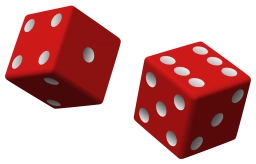# Assume 2

Assume you are working with a standard six-sided die.

What is the probability of rolling the die once and obtaining a 5 and then rolling the die again and obtaining a 2? (input 3 numbers after the decimal, do not round). Report probability as a proportion.

p =  1/36

### Step-by-step explanation:Did you find an error or inaccuracy? Feel free to write us. Thank you!

Tips for related online calculators
Need help calculating sum, simplifying, or multiplying fractions? Try our fraction calculator.
Would you like to compute the count of combinations?